Synchronize limits of multiple axes

## Syntax

``linkaxes(ax)``
``linkaxes(ax,dimension)``

## Description

example

````linkaxes(ax)` synchronizes the limits of the specified vector of axes. Synchronizing limits allows you to zoom or pan in one plot or figure and display the same range of data in another plot or figure. When you first call `linkaxes`, the function chooses new limits that incorporate the current limits of all the specified axes. ```

example

````linkaxes(ax,dimension)` synchronizes the axes limits for the specified axis dimension. For example, `linkaxes(ax,'x')` synchronizes the limits for the x-axis only.```

## Examples

collapse all

Display a tiling of plots using the `tiledlayout` and `nexttile` functions.

Create a 3-by-1 tiled chart layout by using the `tiledlayout` function. Then, create the axes objects `ax1`, `ax2`, and `ax3` by using the `nexttile` function and plot into each axes.

```tiledlayout(3,1) % First plot ax1 = nexttile; x1 = linspace(0,6); y1 = sin(x1); plot(x1,y1) % Second plot ax2 = nexttile; x2 = linspace(0,10); y2 = 2*sin(2*x2); plot(x2,y2) % Third plot ax3 = nexttile; x3 = linspace(0,12,200); y3 = 4*sin(6*x3); plot(x3,y3)```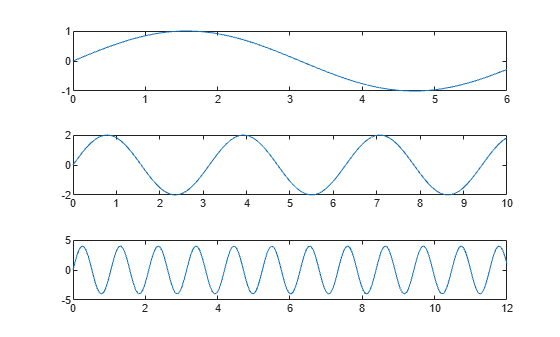Synchronize the x-axis and y-axis limits of each plot. Note that the new axes limits incorporate the old limits.

`linkaxes([ax1 ax2 ax3],'xy')`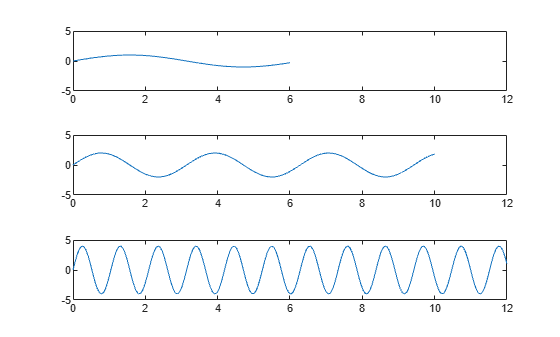Set the x-axis limits for the first plot. All of the axes are linked, so the x-axis limits in the second and third plots also change.

`ax1.XLim = [0 4.5];`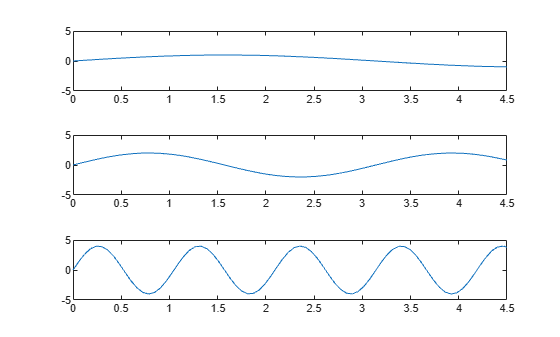Panning or zooming into one of the plots displays the same range of data in the other two plots.

To remove the linking, use `linkaxes([ax1 ax2 ax3],'off')`.

Synchronize and modify the x-axis limits of multiple axes objects by using the `linkaxes` function.

Display a tiling of plots using the `tiledlayout` and `nexttile` functions. Create a 2-by-1 tiled chart layout by using the `tiledlayout` function. Then, create the axes objects `ax1` and `ax2` by using the `nexttile` function and plot into each axes.

```t = tiledlayout(2,1); % First plot ax1 = nexttile; p1 = peaks; surf(ax1,p1); view(3) % Second plot ax2 = nexttile; p2 = peaks(25); mesh(ax2,p2)```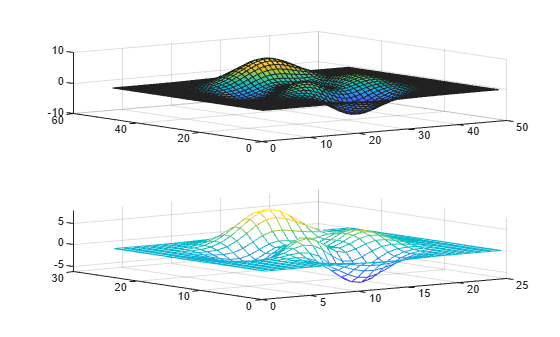Synchronize the x-axis limits for the two axes objects. The x-axis limits for the newly linked axes objects will update to encompass all the data.

`linkaxes([ax1 ax2],'x');`

Update the x-axis limits for all linked axes objects by setting the x-axis limits for just one of the axes objects.

```ax1.XLim = [0 15]; ax2.XLim```
```ans = 1×2 0 15 ```

Remove the linking by turning off synchronization.

`linkaxes([ax1 ax2],'off');`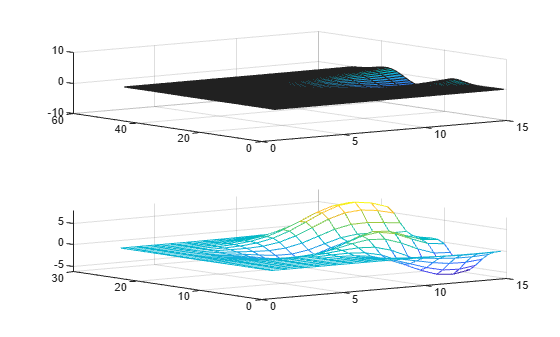When comparing two plots of datetime values in different time zones, set the `ReferenceDate` property of one of the rulers so that both plots reflect the same time zone.

Create a tiling of two plots that compare the number of New Year's Day revelers in London and New York on January 1, 2021. Start by plotting the `London` data in the first tile. By default, the plot uses the time zone of the data.

```London = datetime(2021,1,1,0:17,0,0,"TimeZone","Europe/London"); y = (1./(1:18).^2) * 100000; ax1 = nexttile; semilogy(ax1,London,y) grid on title("New Year's Day Revelers in London")```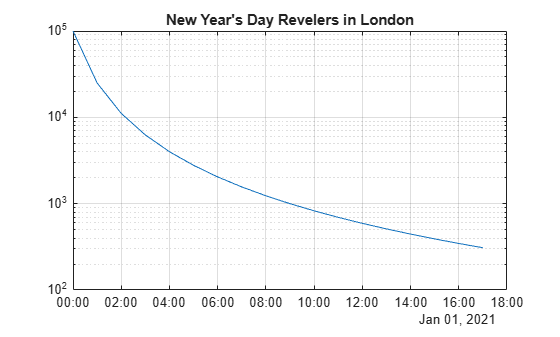Plot the `NY` data. This second plot is in the time zone of New York, so you cannot accurately compare the number of revelers across the two locations at the same point in time by visual inspection.

```NY = datetime(2021,1,1,0:17,0,0,"TimeZone","America/New_York"); ax2 = nexttile; semilogy(ax2,NY,y) grid on title("New Year's Day Revelers in New York")```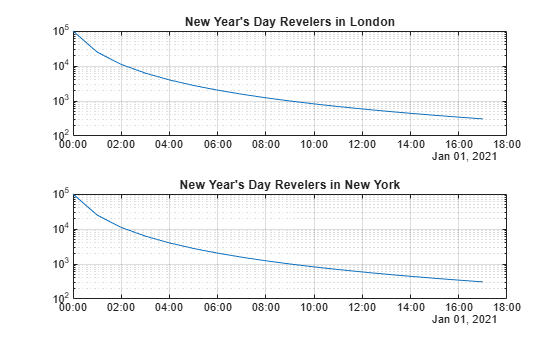Change the time zone for the New York plot by setting the reference date of the x-axis to a datetime value in the time zone of `"Europe/London"`. Then link the two axes. As a result, both plots reflect the same time zone.

```ax2.XAxis.ReferenceDate = datetime(2022,1,1,"TimeZone","Europe/London"); linkaxes([ax1,ax2],"x")```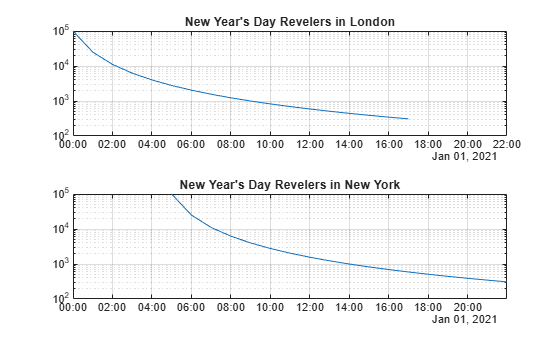## Input Arguments

collapse all

Target axes, specified as a vector of `Axes` objects.

You can link any number of `Axes` objects. For example, `linkaxes([ax1 ax2 ax3])` links `ax1`, `ax2`, and `ax3`. Separate calls to `linkaxes([ax1 ax2])` and `linkaxes([ax2 ax3])` cancels the link between `ax1` and `ax2`.

Axis limits to synchronize, specified as one of these values:

• `'xyz'` — Synchronize the x-axis, y-axis, and z-axis limits.

• `'x'` — Synchronize only the x-axis limits.

• `'y'` — Synchronize only the y-axis limits.

• `'z'` — Synchronize only the z-axis limits.

• `'xy'` — Synchronize only the x-axis and y-axis limits.

• `'xz'` — Synchronize only the x-axis and z-axis limits.

• `'yz'` — Synchronize only the y-axis and z-axis limits.

• `'off'` — Turn off synchronization.

## Version History

Introduced before R2006a

expand all